# Chapter 3 Pulse Code Modulation Pulse Code Modulation

• Slides: 16
Download presentationChapter 3: Pulse Code Modulation Ø Ø Ø Pulse Code Modulation Quantizing Encoding Analogue to Digital Conversion Bandwidth of PCM Signals Huseyin Bilgekul Eeng 360 Communication Systems I Department of Electrical and Electronic Engineering Eastern Mediterranean University 1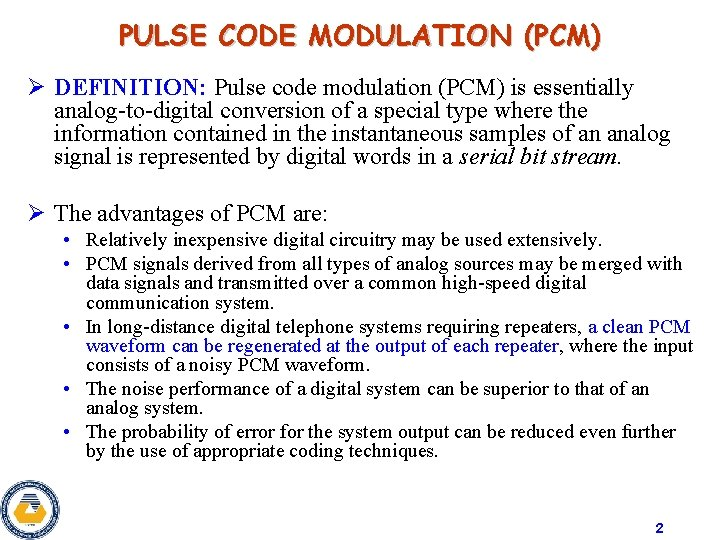PULSE CODE MODULATION (PCM) Ø DEFINITION: Pulse code modulation (PCM) is essentially analog-to-digital conversion of a special type where the information contained in the instantaneous samples of an analog signal is represented by digital words in a serial bit stream. Ø The advantages of PCM are: • Relatively inexpensive digital circuitry may be used extensively. • PCM signals derived from all types of analog sources may be merged with data signals and transmitted over a common high-speed digital communication system. • In long-distance digital telephone systems requiring repeaters, a clean PCM waveform can be regenerated at the output of each repeater, where the input consists of a noisy PCM waveform. • The noise performance of a digital system can be superior to that of an analog system. • The probability of error for the system output can be reduced even further by the use of appropriate coding techniques. 2Sampling, Quantizing, and Encoding Ø The PCM signal is generated by carrying out three basic operations: 1. Sampling 2. Quantizing 3. Encoding 1. 2. Sampling operation generates a flat-top PAM signal. Quantizing operation approximates the analog values by using a finite number of levels. This operation is considered in 3 steps a) Uniform Quantizer b) Quantization Error c) Quantized PAM signal output 3. PCM signal is obtained from the quantized PAM signal by encoding each quantized sample value into a digital word. 3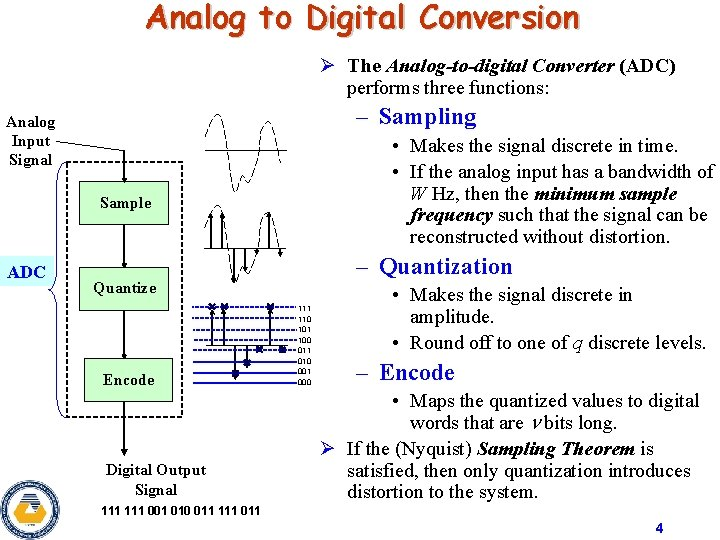Analog to Digital Conversion Ø The Analog-to-digital Converter (ADC) performs three functions: – Sampling Analog Input Signal • Makes the signal discrete in time. • If the analog input has a bandwidth of W Hz, then the minimum sample frequency such that the signal can be reconstructed without distortion. Sample ADC – Quantization Quantize Encode Digital Output Signal 111 110 101 100 011 010 001 000 • Makes the signal discrete in amplitude. • Round off to one of q discrete levels. – Encode • Maps the quantized values to digital words that are bits long. Ø If the (Nyquist) Sampling Theorem is satisfied, then only quantization introduces distortion to the system. 111 001 010 011 111 011 4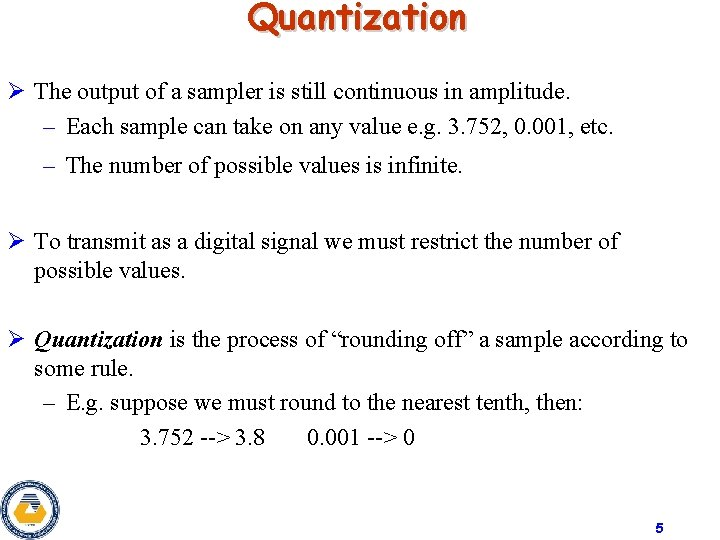Quantization Ø The output of a sampler is still continuous in amplitude. – Each sample can take on any value e. g. 3. 752, 0. 001, etc. – The number of possible values is infinite. Ø To transmit as a digital signal we must restrict the number of possible values. Ø Quantization is the process of “rounding off” a sample according to some rule. – E. g. suppose we must round to the nearest tenth, then: 3. 752 --> 3. 8 0. 001 --> 0 5Illustration of the Quantization Error 6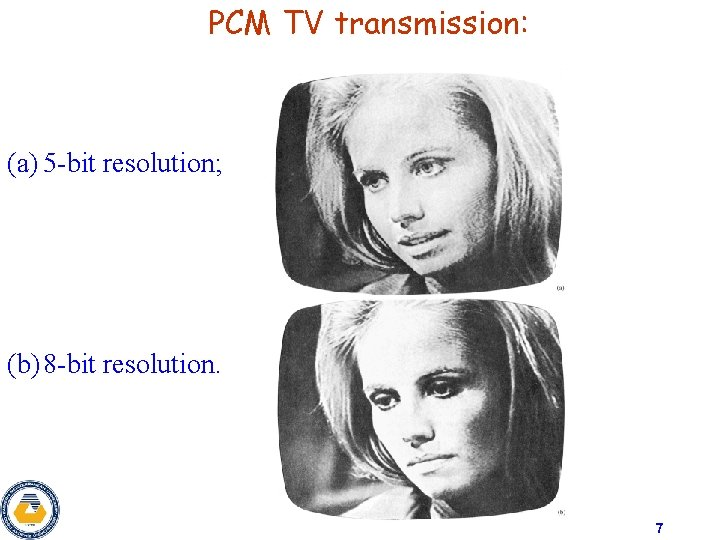PCM TV transmission: (a) 5 -bit resolution; (b) 8 -bit resolution. 7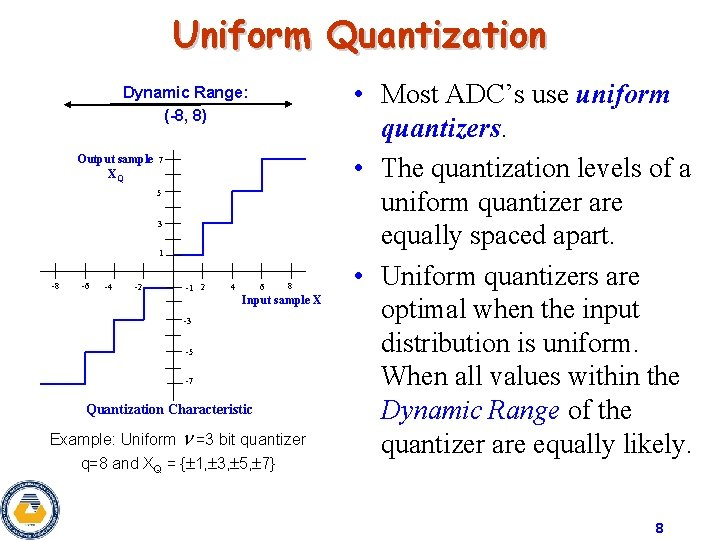Uniform Quantization Dynamic Range: (-8, 8) Output sample XQ 7 5 3 1 -8 -6 -4 -2 -1 2 4 6 8 Input sample X -3 -5 -7 Quantization Characteristic Example: Uniform =3 bit quantizer q=8 and XQ = { 1, 3, 5, 7} • Most ADC’s use uniform quantizers. • The quantization levels of a uniform quantizer are equally spaced apart. • Uniform quantizers are optimal when the input distribution is uniform. When all values within the Dynamic Range of the quantizer are equally likely. 8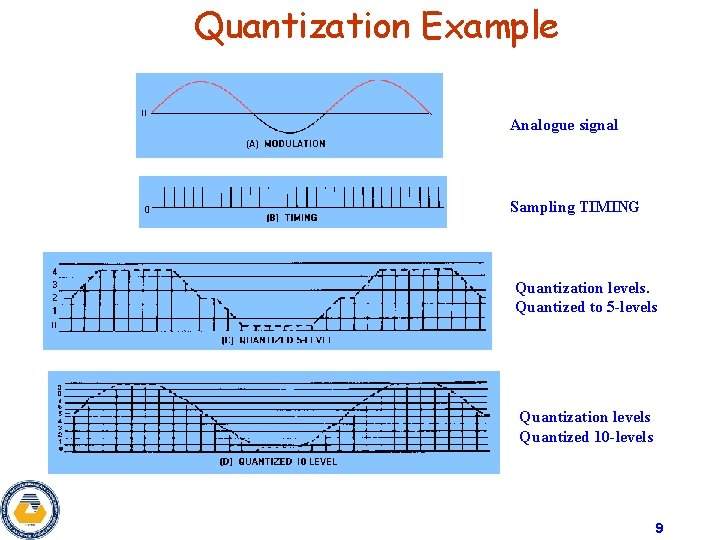Quantization Example Analogue signal Sampling TIMING Quantization levels. Quantized to 5 -levels Quantization levels Quantized 10 -levels 9PCM encoding example Levels are encoded using this table Table: Quantization levels with belonging code words M=8 Chart 1. Quantization and digitalization of a signal. Signal is quantized in 11 time points & 8 quantization segments. Chart 2. Process of restoring a signal. PCM encoded signal in binary form: 101 110 001 010 100 111 100 011 010 101 Total of 33 bits were used to encode a signal 10Encoding • The output of the quantizer is one of M possible signal levels. – If we want to use a binary transmission system, then we need to map each quantized sample into an n bit binary word. • Encoding is the process of representing each quantized sample by an bit code word. – The mapping is one-to-one so there is no distortion introduced by encoding. – Some mappings are better than others. • A Gray code gives the best end-to-end performance. • The weakness of Gray codes is poor performance when the sign bit (MSB) is received in error. 11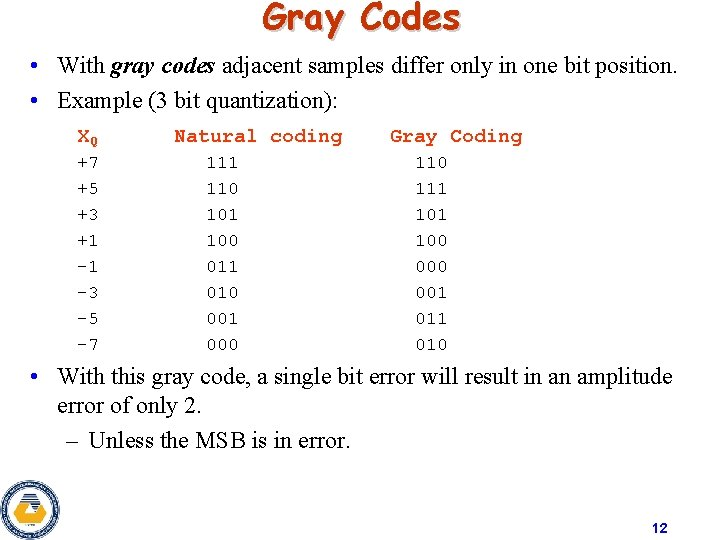Gray Codes • With gray codes adjacent samples differ only in one bit position. • Example (3 bit quantization): XQ +7 +5 +3 +1 -1 -3 -5 -7 Natural coding 111 110 101 100 011 010 001 000 Gray Coding 110 111 100 001 010 • With this gray code, a single bit error will result in an amplitude error of only 2. – Unless the MSB is in error. 12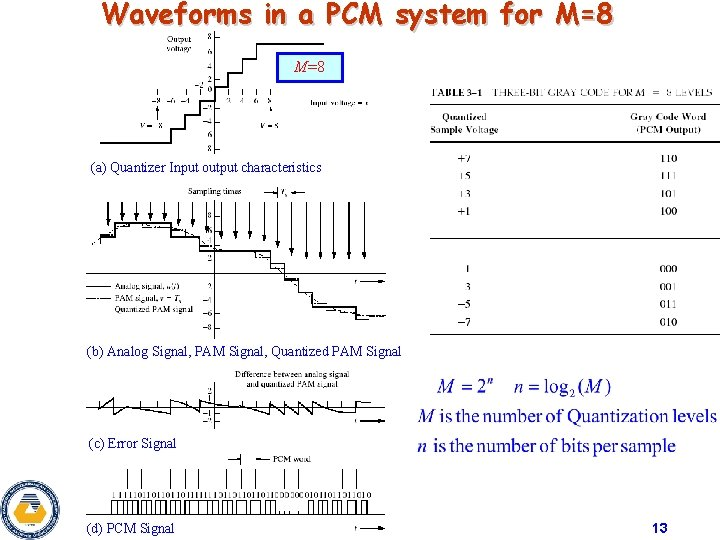Waveforms in a PCM system for M=8 (a) Quantizer Input output characteristics (b) Analog Signal, PAM Signal, Quantized PAM Signal (c) Error Signal (d) PCM Signal 13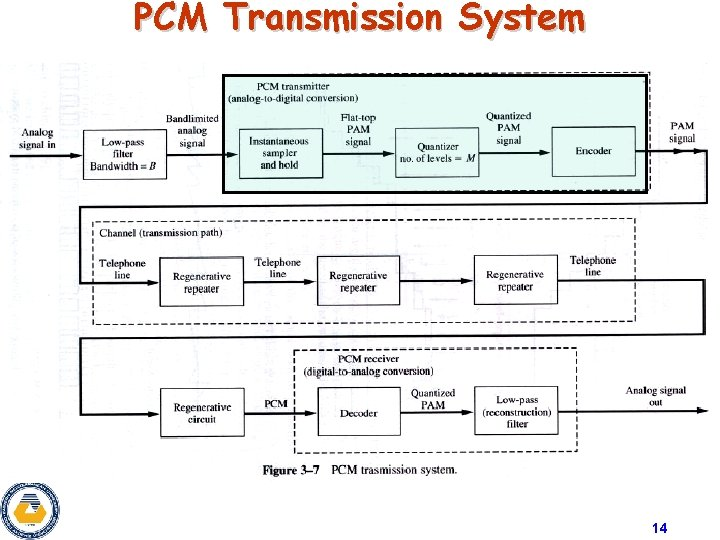PCM Transmission System 14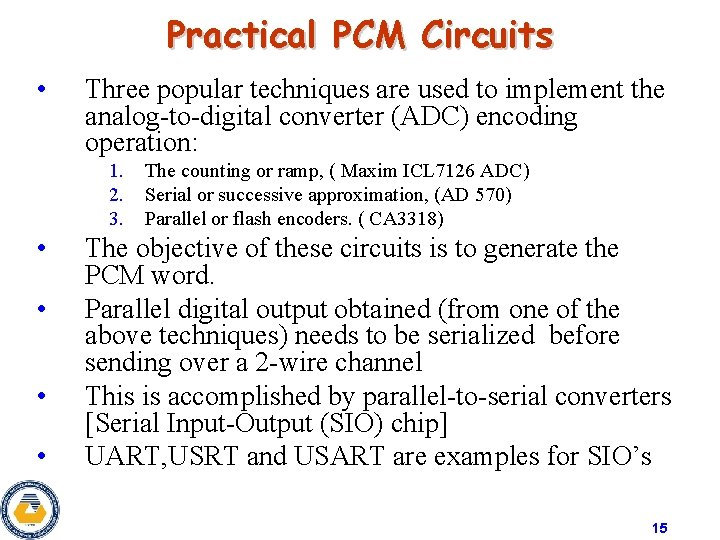Practical PCM Circuits • Three popular techniques are used to implement the analog-to-digital converter (ADC) encoding operation: 1. 2. 3. • • The counting or ramp, ( Maxim ICL 7126 ADC) Serial or successive approximation, (AD 570) Parallel or flash encoders. ( CA 3318) The objective of these circuits is to generate the PCM word. Parallel digital output obtained (from one of the above techniques) needs to be serialized before sending over a 2 -wire channel This is accomplished by parallel-to-serial converters [Serial Input-Output (SIO) chip] UART, USRT and USART are examples for SIO’s 15Bandwidth of PCM Signals • • The spectrum of the PCM signal is not directly related to the spectrum of the input signal. The bandwidth of (serial) binary PCM waveforms depends on the bit rate R and the waveform pulse shape used to represent the data. The Bit Rate R is R=nfs Where n is the number of bits in the PCM word (M=2 n) and fs is the sampling rate. For no aliasing case (fs≥ 2 B), the MINIMUM Bandwidth of PCM Bpcm(Min) is: Bpcm(Min) = R/2 = nfs//2 The Minimum Bandwidth of nfs//2 is obtained only when sin(x)/x pulse is used to generate the PCM waveform. • For PCM waveform generated by rectangular pulses, the First-null Bandwidth is: Bpcm = R = nfs 16